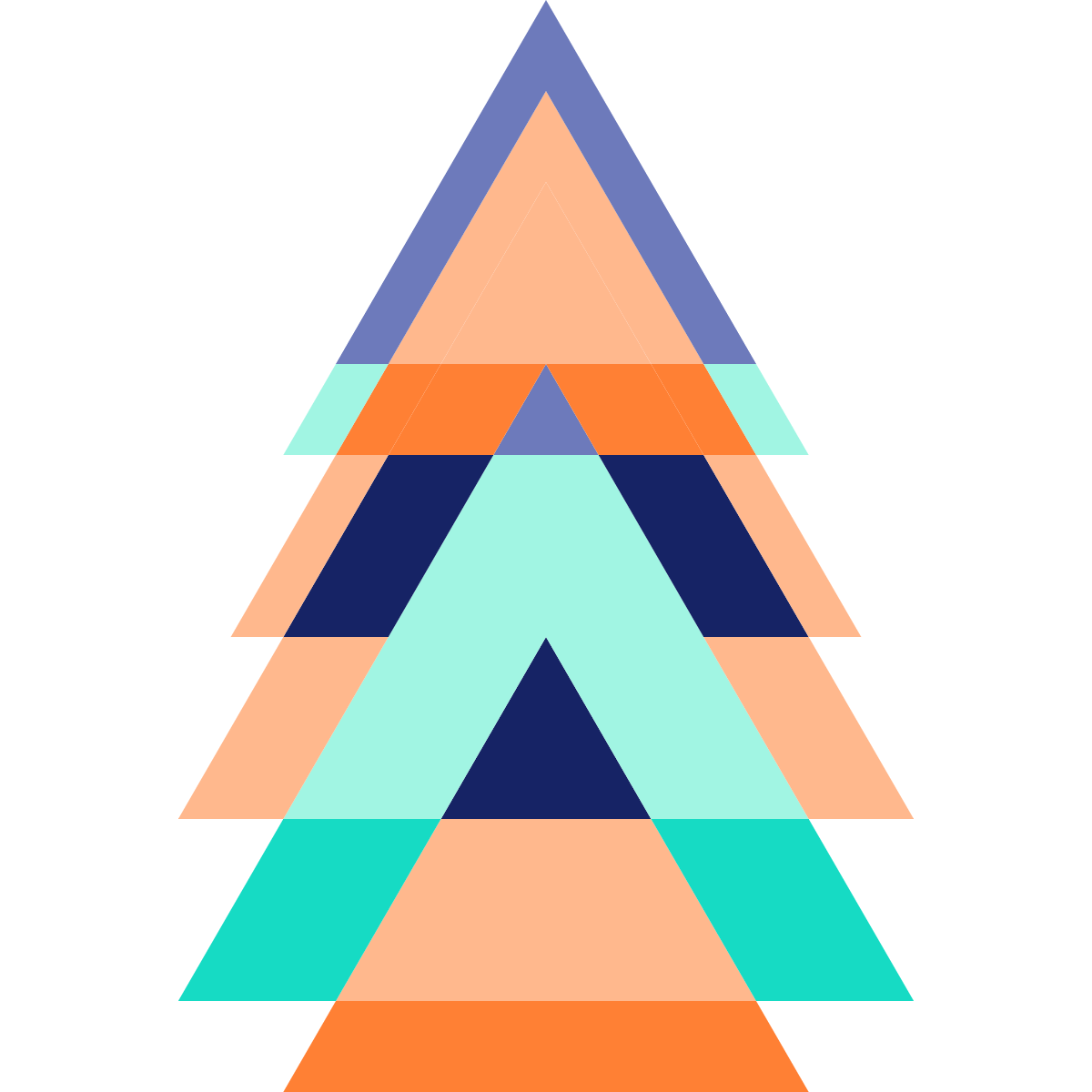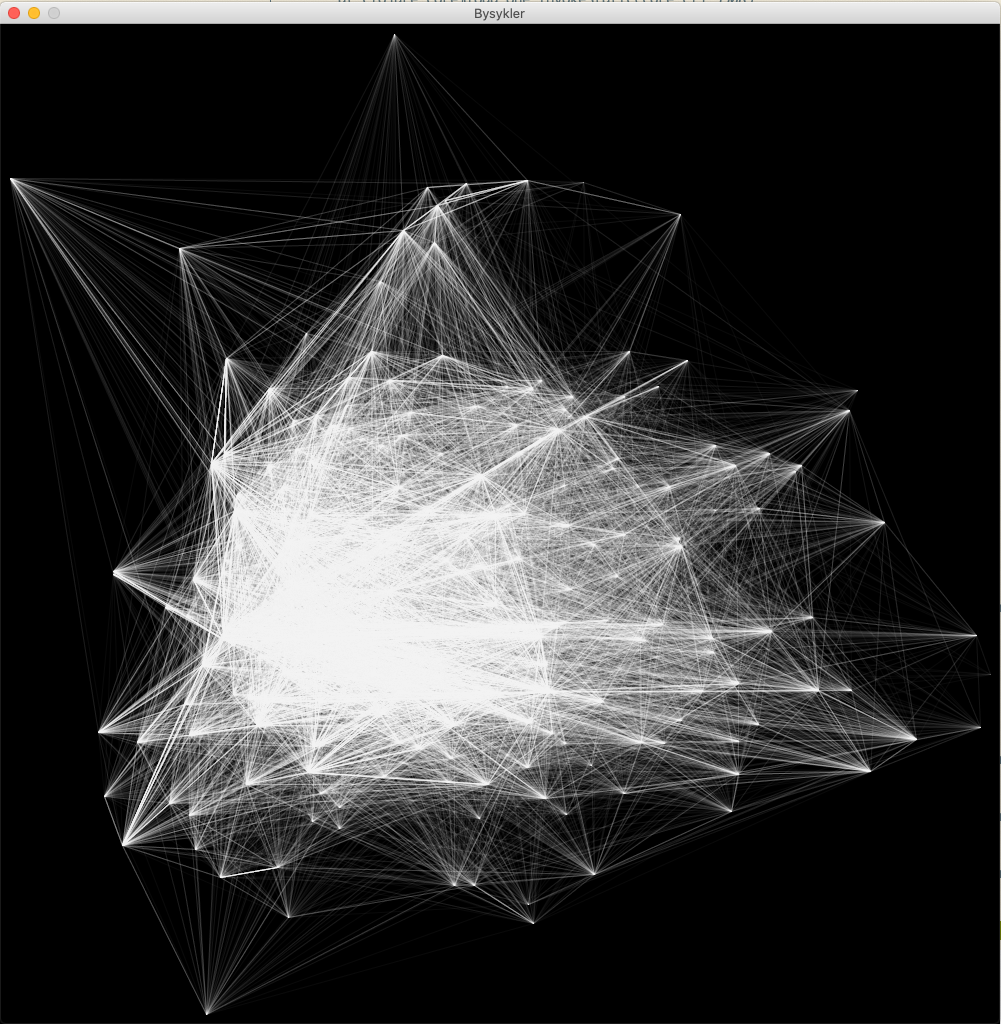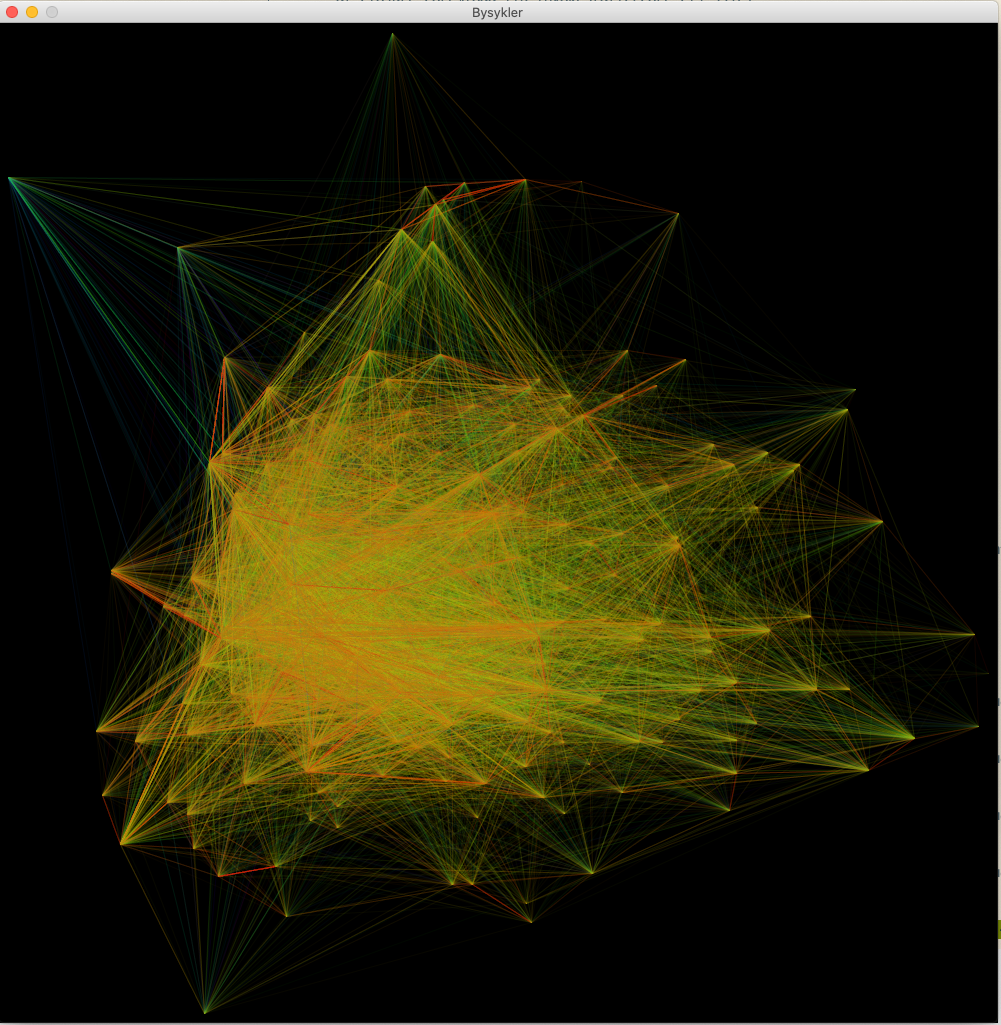# Visualize bike rental data in Clojure

A 5 minute read written by
Fredrik Meyer
17.12.2019

This will be a short post on how to visualize bike rental data in Clojure. Let's get to it!

## Starting coding

I'll assume you know how to install programs on your computer. You will need Clojure (`brew install clojure` on a Mac), and Leiningen, our package managing system (`brew install leiningen`). You will also have to have Java installed on your system.

To get something running, write `lein new app city-bikes` in your terminal. This will create a folder named `city-bikes` with a template project. To test that everything works correctly, `cd` into your folder and type `lein run`. Hopefully, you'll see `Hello, World!` printed out.

Now we're ready to start coding!

First of all, we need some data to work with. We'll use open data provided by Oslo Citybikes. Download one of the JSON files containing bike rental data and put it in the root folder. Rename it to `city-bikes.json`.

We'll use Quil to visualize the data. It is a wrapper of Processing written for Clojure. To use Quil, we must add it as a dependency in our project. We will also need to process some JSON, so we'll add a dependency to the `org.clojure/data.json` library. To do that, update the `project.clj` file to look like this:

``````(defproject bysykler "0.1.0-SNAPSHOT"
:description "FIXME: write description"
:url "http://example.com/FIXME"
:license {:name "EPL-2.0 OR GPL-2.0-or-later WITH Classpath-exception-2.0"
:url "https://www.eclipse.org/legal/epl-2.0/"}
:dependencies [[org.clojure/clojure "1.10.0"]
[org.clojure/data.json "0.2.7"]
[quil "3.1.0"]]
:main ^:skip-aot bysykler.core
:target-path "target/%s"
:profiles {:uberjar {:aot :all}})``````

To download the dependencies, run `lein deps` in your terminal. To be able to use the dependencies, we must import them. Update the namespace declaration:

``````(ns bysykler.core
(:require [quil.core :as q]
[clojure.data.json :as json]))``````

Let us take a look at the data: first of all we'll take a look at how the data looks. Using for example `jq`:

``````[~/code/bysykler]\$ jq '.' bysykler.json
{
"started_at": "2019-06-01 00:26:52.688000+00:00",
"ended_at": "2019-06-01 00:36:06.407000+00:00",
"duration": 553,
"start_station_id": "739",
"start_station_name": "Hammersborg Torg",
"start_station_description": "i Møllergata",
"start_station_latitude": 59.916536,
"start_station_longitude": 10.749162,
"end_station_id": "585",
"end_station_name": "Nordre gate",
"end_station_description": "Øst for Grünerbrua",
"end_station_latitude": 59.9212171,
"end_station_longitude": 10.7547918
}``````

For this visualization, we're only interested in the start- and end-points and the duration of each bicycle trip.

We'll write a small Clojure function that takes a Clojure map and returns only the interesting keys (to avoid clutter when debugging, for example):

``````(defn keep-interesting [b]
(select-keys b
[:start_station_latitude
:start_station_longitude
:end_station_latitude
:end_station_longitude
:duration]))``````

Now we can read the data into a Clojure variable:

``````(def bysykler
(let [parsed (-> "city-bikes.json"
slurp
(map keep-interesting parsed)))``````

Notice how we chain the computation: we start with an input, the name of the file we're interested in, and then we pass it to one function after another: first `slurp` the file (reading it as a string), and then parse it as a JSON. The result is a long list, over which we map the `keep-interesting` function.

To test how this works, try adding the following in your `core.clj` function:

``(println (first bysykler))``

After running `lein deps && lein run`, you should see the data of the first bicycle trip printed.

# Visualize something

Now to the fun part! First we must setup Quil. Quil need a `setup` function, and a `draw` function. Add the following functions to `core.clj`:

``````(defn setup []
(q/color-mode :hsb 100 100 100)
(q/background 0.)
(q/stroke 100. 10)
(q/stroke-weight 1))``````

and

``````(q/defsketch quil-drawings
:title "Bysykler"
:size [1000 1000]
:setup setup
:draw draw
:features [:keep-on-top :no-bind-output])``````

and

``````(defn draw []
(q/ellipse 500 500 300 300)
(q/no-loop))``````

Save, and run `lein run`. If everything works, you should see a big circle in the middle of the picture.

But we really want to plot bike rental data. We'll keep it simple and draw lines from the start position of the trip to the end position. We are given latitude and longitude, but want to draw them on a screen. A really simple way to do this, is to think of latitude and longitude as x- and y-coordinates. This works for small maps, because the Earth is approximately flat:

``````(def max-start-lat (apply max (map :start_station_latitude bysykler)))
(def max-start-lon (apply max (map :start_station_longitude bysykler)))
(def min-start-lat (apply min (map :start_station_latitude bysykler)))
(def min-start-lon (apply min (map :start_station_longitude bysykler)))

(defn lat-long-to-xy [lat lon]
[
(q/map-range lat min-start-lat max-start-lat 10 (- 1000 10))
(q/map-range lon min-start-lon max-start-lon 10 (- 1000 10))
])``````

The above code snippet first finds the maximum and minimum latitudes and longitudes of all the trips, and then we normalize it to lie inside the canvas. We now have all we need top draw the trips. Update your draw function as so:

``````(defn draw []
(doseq [b bysykler]
(let [[x y] (lat-long-to-xy (:start_station_latitude b) (:start_station_longitude b))
[aa bb] (lat-long-to-xy (:end_station_latitude b) (:end_station_longitude b))
]
(q/line x y aa bb)))
(q/no-loop))``````

If you now run `lein run`, and everything is correct, you'll see a nice black and white visualization of all the trips. This is where it was nice that we wrote `(q/stroke 100. 10)` in the `setup` function: all lines drawn have very low opacity, so we can draw the same trip several times to increase intensity.Finally, we'll add some color. We'll color the trips based on its duration. The following code maps all the durations and maps them to the interval 0-10.

``````(def min-dur (apply min (map :duration bysykler)))
(def max-dur (apply max (map :duration bysykler)))
(defn normalize-duration [dur]
(q/map-range dur min-dur 500 0 10))``````

``````(defn draw []
(println (first bysykler))
(doseq [b bysykler]
(let [[x y] (lat-long-to-xy (:start_station_latitude b) (:start_station_longitude b))
[aa bb] (lat-long-to-xy (:end_station_latitude b) (:end_station_longitude b))
dur (normalize-duration (:duration b))
]
(q/stroke dur 100 80 10)
(q/line x y aa bb)))
(q/no-loop))``````

Notice how now each line has a different stroke depending on the duration.

Running, you should see something like this:If you don't want to type all the code yourself, you can check out this Github repository, where I've uploaded the code. Clone it, and run `lein run`!